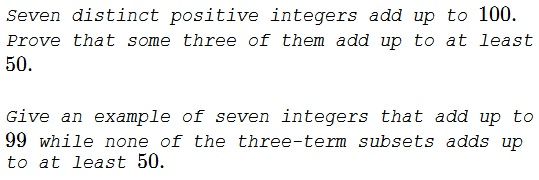# Seven Integers That Add Up to 100

### Problem### Solution 1

Let's order the seven integers in increasing order $a_1\lt a_2\lt a_3\lt a_4\lt a_5\lt a_6\lt a_7$ and consider the sum of the largest three: $a_5+a_6+a_7.$ We'll prove that $a_5+a_6+a_7\ge 50.$

If $a_4\ge 15,$ then $a_5+a_6+a_7\ge 16+17+18=51.$

If $a_4\lt 15,$ then $a_4+a_3+a_2+a_1\lt 14+13+12+11=50,$ so that $a_5+a_6+a_7\ge 100-50=50.$

The following supplies a counterexample of the seven integers that add up to $99:$

$11+12+13+14+15+16+18=99,$

while the largest subsum, $15+16+18=49\lt 50.$

### Solution 2

Let the seven numbers that sum to $100$ be $a_1\lt a_2\lt \ldots\lt a_7$.

Suppose no three of the seven numbers add up to a number $\geq 50$. Thus, $a_5+a_6+a_7\lt 50$, i.e., $a_1+a_2+a_3+a_4\gt 50$. The smallest possible $a_4$ that satisfies this condition is the smallest $a_4$ that satisfies the condition

$(a_4-3)+(a_4-2)+(a_4-1)+a_4\gt 50$

or $a_4=15$. Hence, $a_5,a_6,a_7\gt 15$. The smallest sum $a_5+a_6+a_7$ is thus $16+17+18=51$. This contradicts our supposition. Thus, there is atleast one set of three numbers out of the seven that add up to at least $50$.

For seven numbers adding to $99$, let $a_7=18,~a_6=16,~a_5=15$ (to maximize the sum to $49).$ Thus, $a_1+a_2+a_3+a_4=50.$ Let $a_4=14,~a_3=13,~a_2=12,~a_1=11.$

### Acknowledgment

This is a problem from the book Mathematical Olympiad Challenges by T. Andreescu and Răzvan Gelca (Burkhäuser, 2004, p 60).

Solution 2 is by Amit Itagi.